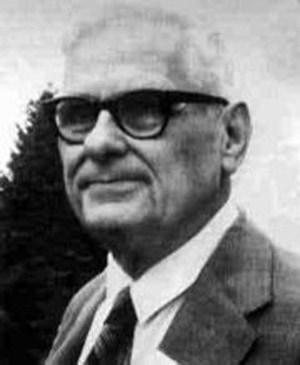A# Church, Alonzo (1903–1995)Alonzo Church was an American logician and professor at Princeton University who was an early pioneer of theoretical computer science. He is best known for his development, in 1934, of the so-called lambda calculus, a model of computation, and his discovery, in 1936, of an "undecidable problem" within it. This result preceded Alan Turing's famous work on the halting problem, which also pointed out the existence of a problem unsolvable by mechanical means. Church and Turing then showed that the lambda calculus and the Turing machine, which is used in the halting problem, are equivalent in capability; they also demonstrated a variety of alternative "mechanical processes for computation" with equivalent computational abilities.

## Church-Turing thesis

The Church-Turing thesis is a logical/mathematical postulate, independently arrived at by Alan Turing and Alonzo Church, which asserts that as long as a procedure is sufficiently clear-cut and mechanical, there is some algorithmic way of solving it (such as via lambda calculus or computation on a Turing machine). Thus, there are some processes or problems that are computable according to some set of algorithms, and other processes or problems that are not. A strong form of the Church-Turing thesis claims that all neural and psychological processes can be simulated as computational processes on a computer.

## Lambda calculus

Lambda calculus is a construction in abstract logic created by Church in the 1930s that has had practical application in the design of programming languages, most notably LISP, which was inspired by it. Lambda calculus turns out to be a model of computation that is capable of universal computation. It involves the evaluation of lambda expressions, which are definitions of unnamed functions. The format of a lambda expression is:

lambda [parameter list] . [body of function]

where the body of a function is just the rules for computing the function's value. For example, the function of taking the square of a number is expressed in lambda notation as:

lambda x . x*x

Originally the notation involved the use of a "^" above the first parameter but this was typographically inconvenient and so the "^" was replaced by the Greek letter Λ which roughly resembles a "^". Of course the Λ must be placed before the first parameter rather than above it.

It may seem puzzling upon encountering the lambda notation in LISP as to why it is needed. The lambda notation is essential for several reasons. First there is a need for a means of defining a function without giving it a name. Naming functions is an easy task but there is an arbitrariness to choosing a name that does not fit in well with computer processing. Functions could be named serially in the order in which they arise in a program. This is feasible but awkward. Often there is not real need for a function to have a name. So the lambda notation handles the problem of creating functions without naming them. Of course functions can be given names to facilitate reference to them if that is needed.

The power of the lambda notation is in its generality. The lambda notation will handle the case in which the value of a function is a function. In many computer languages the value of a function must be an element of a set, such as a number or a string or the label of a function. In the lambda notation the value can be a function, not the name or label of a function but a function itself.